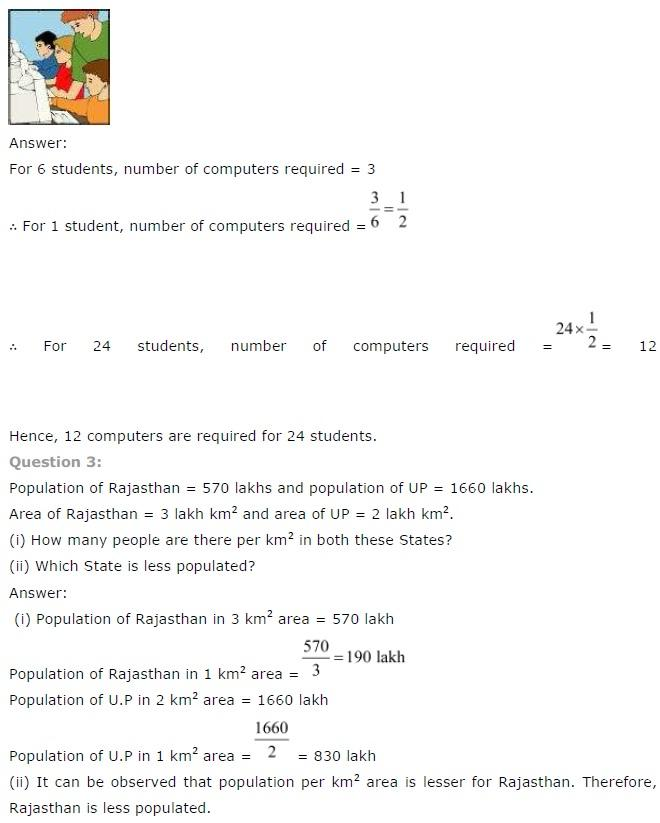# NCERT Solutions class 7 Maths Chapter-8 Exercise 8.1

NCERT Solutions Class-7 Maths chapter-8 Comparing Quantities  Exercise-8.1 is prepared by academic team of pw all the questions of NCERT text book are solved step by step with proper and detail solutions explaining each and every questions . For More and additional questions of CBSE class 7 maths you can go to class 7 maths sections. NCERT class 7 Maths Solutions is the best way to enhanced your mathematics skill. And pw practice worksheet & question bank will help you a lot .

## NCERT Solutions class 7 Maths Chapter-8 Comparing Quantities

### Solutions of Chapter Comparing Quantities  Exercise-8.1

Question 1:
Find ratio of:
(a) Rs. 5 to 50 paise
(b) 15 kg to 210 g
(c) 9 m to 27 cm
(d) 30 days to 36 hours

(a) Rs. 5 to 50 paise
We have,
Rs 5 to 50 Paise
We know that,
1 Rupee = 100 paise
Therefore,
5 Rupees = 500 Paise
Hence,
Ratio = Rs 5/50 Paise
= 500/50
= 10/1
Therefore,
Required ratio = 10: 1

(b) 15 kg to 210 g
We have,
15 kg to 210 g
We know that,
1 kg = 1000 g
Therefore,
15 kg = 15000 g
Hence,
Ratio = 15 kg/210 g
= 15000/210
= 500/7
Therefore,
Required ratio = 500: 7

(c) 9 m to 27 cm
We have,
9 m to 27 cm
We know that,
1 m = 100 cm
Therefore,
9 m = 900 cm
Hence,
Required ratio = 9 m/27 cm
= 900/27
= 100/3
Therefore,
Required ratio = 100: 3

(d) 30 days to 36 hours
We have,
30 days to 36 hours
We know that,
1 day = 24 hours
Therefore,
30 days = 30 × 24
= 720 hours
Hence,
Required ratio = 30 days/36 hours
= 720/36
= 20/1
Therefore,
Required ratio = 20: 1

Question 2:
In a computer lab, there are 3 computers for every 6 students.  How many computers will be needed for 24 students?NCERT CLASS 7 MATHEMATICS SOLUTIONS